# Class operators class polynomial protected int n double

• Slides: 21Class operatorsclass polynomial { protected: int n; double *a; public: polynomial(){}; …. . ; // constructor ~polynomial(); // destructor //member functions. . . double f (const double) const; // polynomial value // friend functions and operators friend polynomial operator+ (const polynomial, const polynomial); friend polynomial operator- (const polynomial, const polynomial); friend polynomial operator* (const double, const polynomial); }; // end of class definition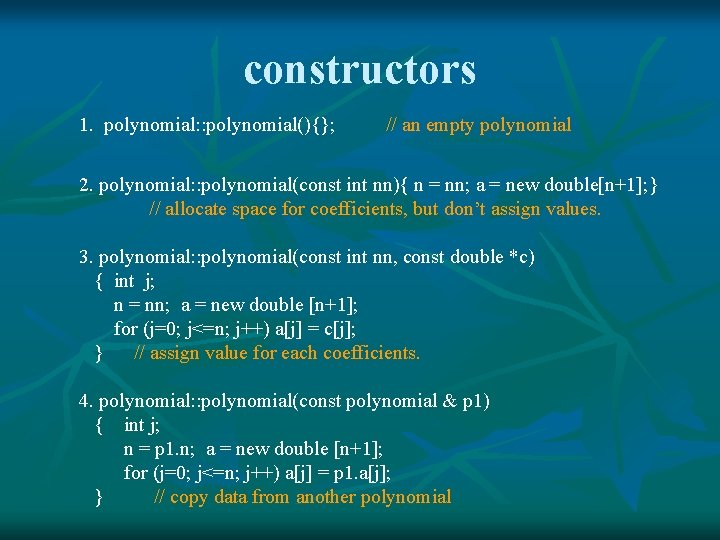constructors 1. polynomial: : polynomial(){}; // an empty polynomial 2. polynomial: : polynomial(const int nn){ n = nn; a = new double[n+1]; } // allocate space for coefficients, but don’t assign values. 3. polynomial: : polynomial(const int nn, const double *c) { int j; n = nn; a = new double [n+1]; for (j=0; j<=n; j++) a[j] = c[j]; } // assign value for each coefficients. 4. polynomial: : polynomial(const polynomial & p 1) { int j; n = p 1. n; a = new double [n+1]; for (j=0; j<=n; j++) a[j] = p 1. a[j]; } // copy data from another polynomial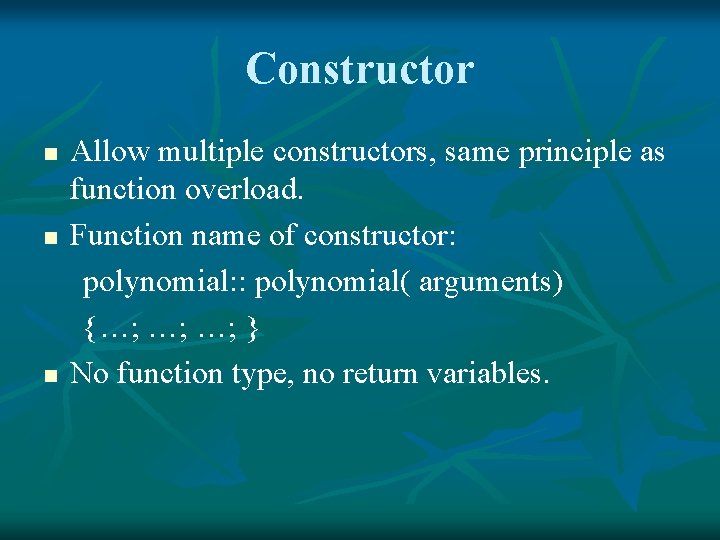Constructor n n n Allow multiple constructors, same principle as function overload. Function name of constructor: polynomial: : polynomial( arguments) {…; …; …; } No function type, no return variables.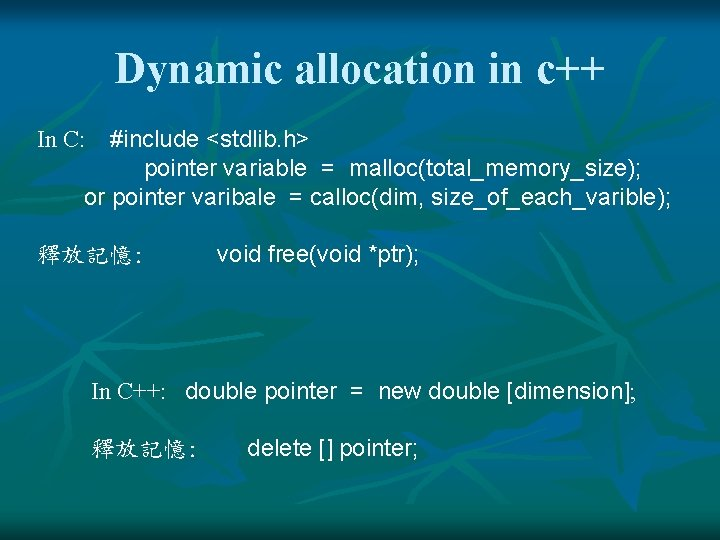Dynamic allocation in c++ #include <stdlib. h> pointer variable = malloc(total_memory_size); or pointer varibale = calloc(dim, size_of_each_varible); In C: 釋放記憶: void free(void *ptr); In C++: double pointer = new double [dimension]; 釋放記憶: delete [] pointer;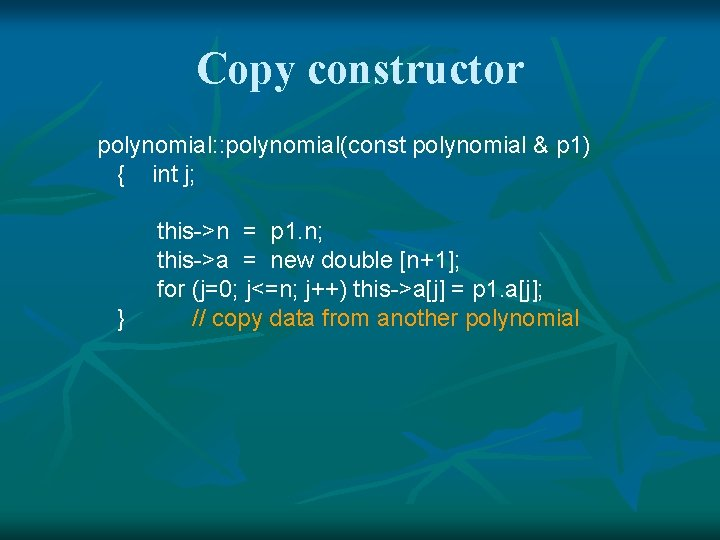Copy constructor polynomial: : polynomial(const polynomial & p 1) { int j; } this->n = p 1. n; this->a = new double [n+1]; for (j=0; j<=n; j++) this->a[j] = p 1. a[j]; // copy data from another polynomial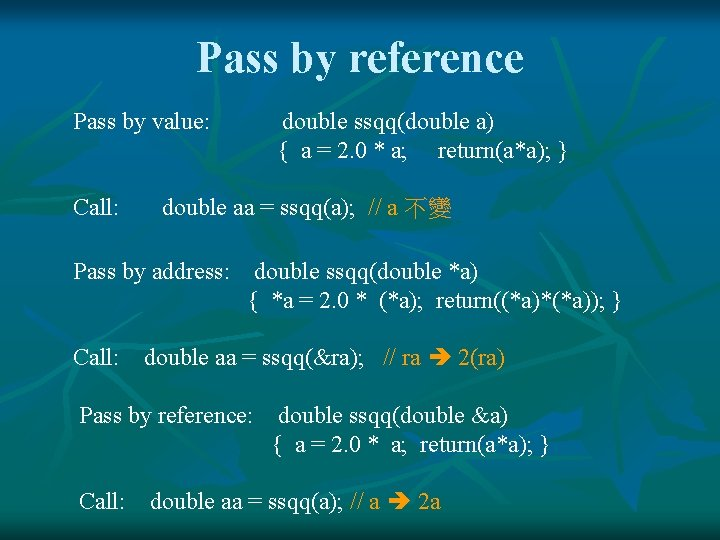Pass by reference Pass by value: Call: double ssqq(double a) { a = 2. 0 * a; return(a*a); } double aa = ssqq(a); // a 不變 Pass by address: double ssqq(double *a) { *a = 2. 0 * (*a); return((*a)*(*a)); } Call: double aa = ssqq(&ra); // ra 2(ra) Pass by reference: Call: double ssqq(double &a) { a = 2. 0 * a; return(a*a); } double aa = ssqq(a); // a 2 aDeconstructor class polynomial { protected: int n; double *a; public: …; …; ~polynomial{ delete [] this->a; } }; polynomial p 1(5, acoef); // 宣告 5 階多項式 p 1. ~polynomial(); // 釋放 p 1. a 的記憶空間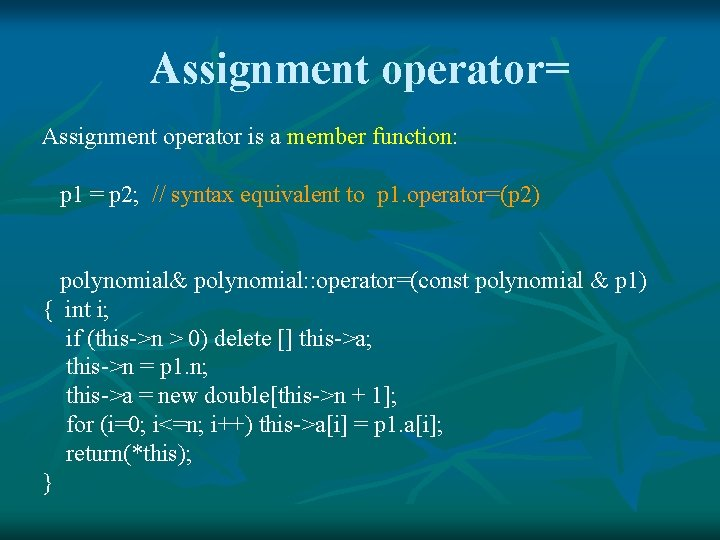Assignment operator= Assignment operator is a member function: p 1 = p 2; // syntax equivalent to p 1. operator=(p 2) polynomial& polynomial: : operator=(const polynomial & p 1) { int i; if (this->n > 0) delete [] this->a; this->n = p 1. n; this->a = new double[this->n + 1]; for (i=0; i<=n; i++) this->a[i] = p 1. a[i]; return(*this); }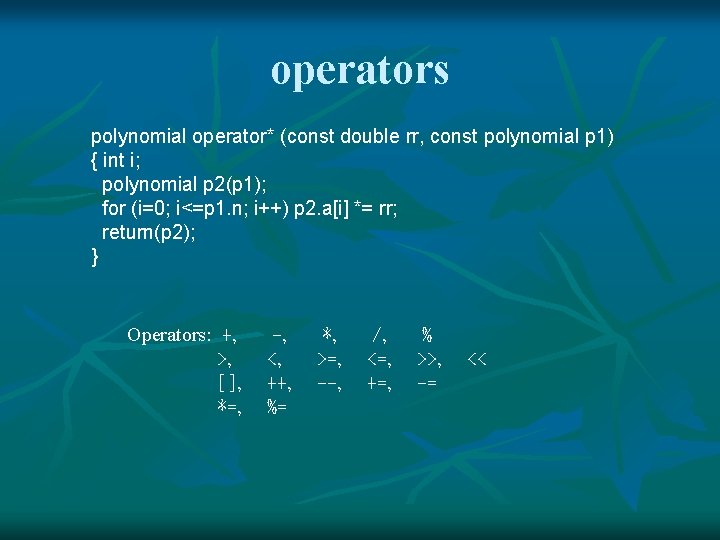operators polynomial operator* (const double rr, const polynomial p 1) { int i; polynomial p 2(p 1); for (i=0; i<=p 1. n; i++) p 2. a[i] *= rr; return(p 2); } Operators: +, >, [], *=, -, <, ++, %= *, >=, --, /, <=, +=, % >>, -= <<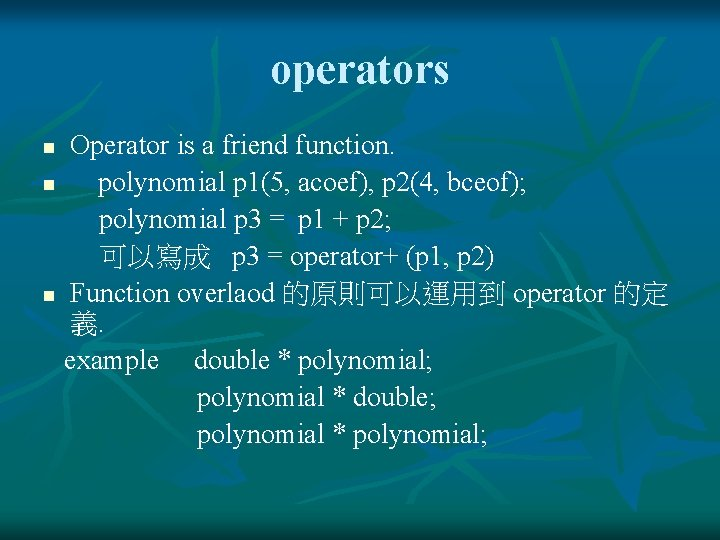operators Operator is a friend function. n polynomial p 1(5, acoef), p 2(4, bceof); polynomial p 3 = p 1 + p 2; 可以寫成 p 3 = operator+ (p 1, p 2) n Function overlaod 的原則可以運用到 operator 的定 義. example double * polynomial; polynomial * double; polynomial * polynomial; nPolynomial operator + polynomial operator+(polynomial p 1, polynomial p 2) { int i; if (p 1. n > p 2. n) { polynomial pp(p 1); for (i=0; i<=p 2. n; i++) pp. a[i] += p 2. a[i]; return(pp); } else { polynomial pp(p 2); for (i=0; i<=p 1. n; i++) pp. a[i] += p 1. a[i]; return(pp); } }polynomial operator if (p 1. n > p 2. n) { polynomial pp(p 1); for (i=0; i<=p 2. n; i++) pp. a[i] -= p 2. a[i]; return(pp); } else { polynomial pp(p 2); pp = (-1. 0) * pp; for (i=0; i<=p 1. n; i++) pp. a[i] += p 1. a[i]; return(pp); }Polynomial operator* polynomial operator* (const polynomial p 1, const polynomial p 2) { int i, j, n 3=p 1. n+p 2. n; double sum; polynomial p 3(n 3); for (i=0; i<=n 3; i++) { sum = 0. 0; for (j=0; j<=i; j++) if ((j<=p 1. n) && (i-j)<= p 2. n) sum += (p 1. a[j]*p 2. a[i-j]); p 3. a[i] = sum; } return(p 3); }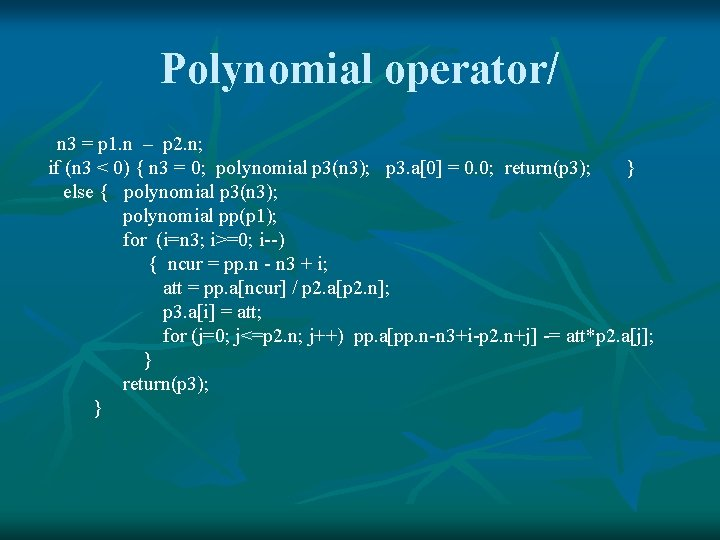Polynomial operator/ n 3 = p 1. n – p 2. n; if (n 3 < 0) { n 3 = 0; polynomial p 3(n 3); p 3. a = 0. 0; return(p 3); } else { polynomial p 3(n 3); polynomial pp(p 1); for (i=n 3; i>=0; i--) { ncur = pp. n - n 3 + i; att = pp. a[ncur] / p 2. a[p 2. n]; p 3. a[i] = att; for (j=0; j<=p 2. n; j++) pp. a[pp. n-n 3+i-p 2. n+j] -= att*p 2. a[j]; } return(p 3); }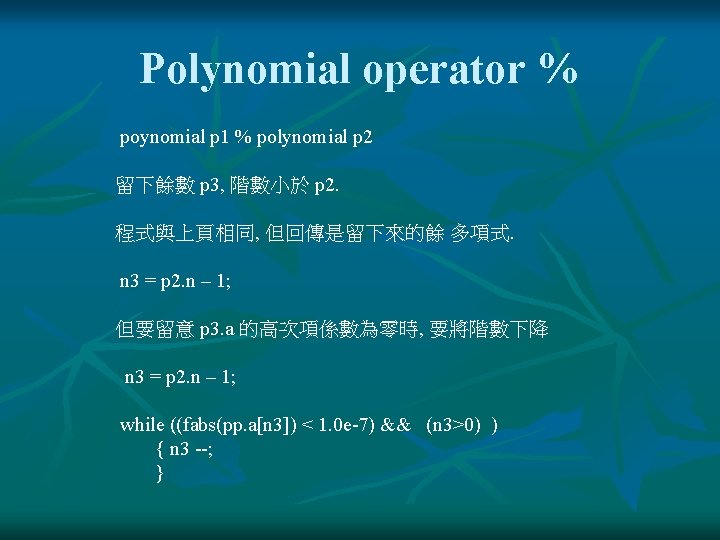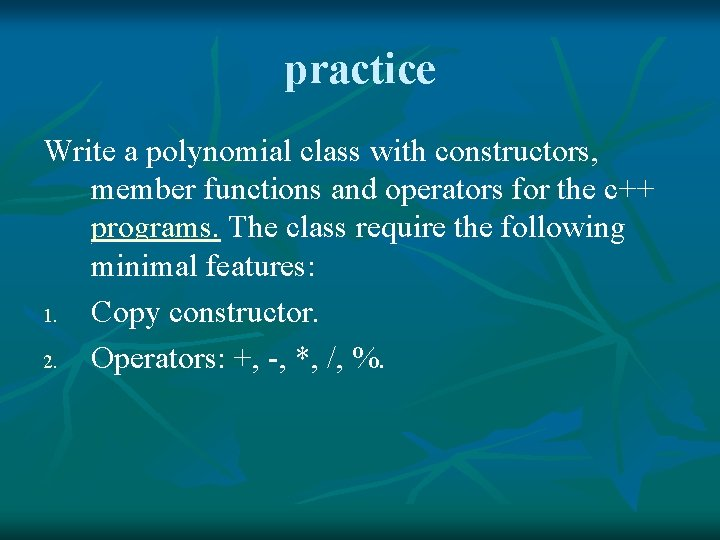practice Write a polynomial class with constructors, member functions and operators for the c++ programs. The class require the following minimal features: 1. Copy constructor. 2. Operators: +, -, *, /, %.Class Matrix class Matrix { protected: int nrow, ncol; double *xpt; public: constructors; member_functions; friend_operators; }; nrow: number of rows ncol: number of columns xpt : pointer for memory reallocation for matrix elements Example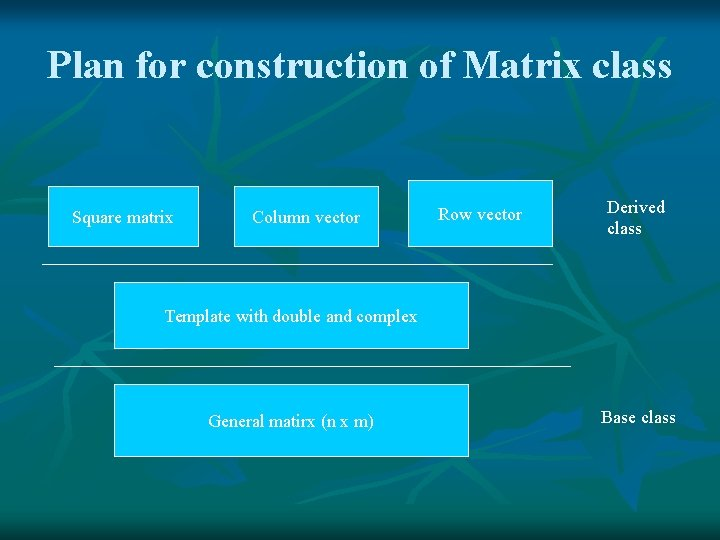Plan for construction of Matrix class Square matrix Column vector Row vector Derived class Template with double and complex General matirx (n x m) Base class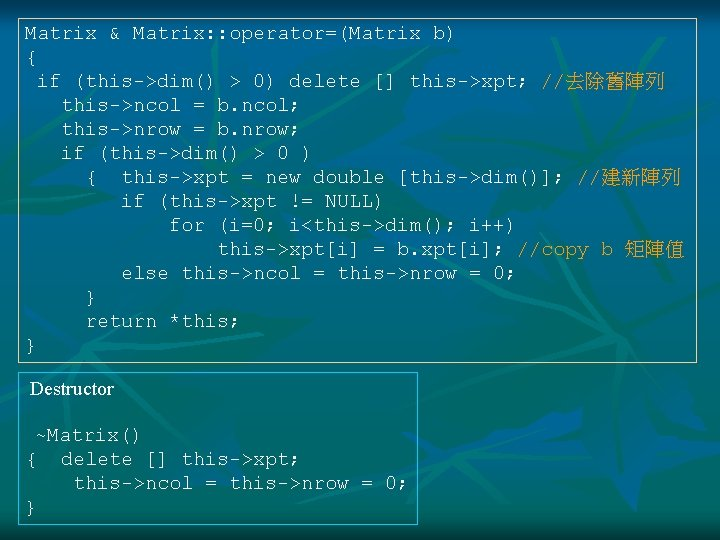Matrix & Matrix: : operator=(Matrix b) { if (this->dim() > 0) delete [] this->xpt; //去除舊陣列 this->ncol = b. ncol; this->nrow = b. nrow; if (this->dim() > 0 ) { this->xpt = new double [this->dim()]; //建新陣列 if (this->xpt != NULL) for (i=0; i<this->dim(); i++) this->xpt[i] = b. xpt[i]; //copy b 矩陣值 else this->ncol = this->nrow = 0; } return *this; } Destructor ~Matrix() { delete [] this->xpt; this->ncol = this->nrow = 0; }